# Morphism

(diff) ← Older revision | Latest revision (diff) | Newer revision → (diff)

of a category

A term used to denote the elements of an arbitrary category which play the role of mappings of one set into another, homomorphisms of groups, rings, algebras, continuous mappings of topological spaces, etc. A morphism of a category is an undefined concept. Each category consists of elements of two classes, called the class of objects and the class of morphisms, respectively. The class of morphisms of a categoryis usually denoted by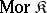.

Any morphismof a category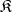has a uniquely defined domain (source)and a uniquely defined codomain (target). All morphisms with common domainand codomainform a subsetof. The fact thathas domainand codomaincan be written in the usual way: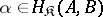or, using arrows,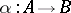,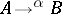, etc.

The division of the elements of a category into morphisms and objects is meaningful only within the context of a fixed category, since the morphisms of one category may be the objects of another and conversely. The morphisms of any category form a system that is closed under a partial binary operation — multiplication. Depending on the properties of morphisms relative to this operation, special classes of morphisms can be distinguished, for example, the classes of monomorphisms, epimorphisms, bimorphisms, isomorphisms, null (zero) morphisms, normal monomorphisms, normal epimorphisms, etc. (cf. Monomorphism; Epimorphism; Bimorphism; Isomorphism; Normal monomorphism; Normal epimorphism).